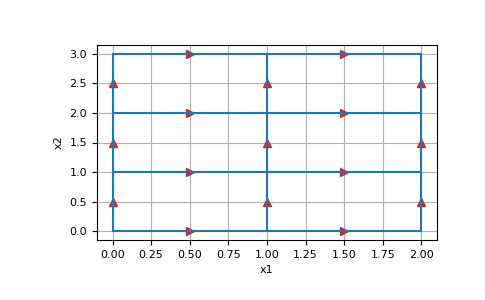# discretize.base.BaseTensorMesh.n_edges_per_direction¶

property BaseTensorMesh.n_edges_per_direction

The number of edges in each direction

This property returns a tuple with the number of edges in each axis direction of the mesh. For a 3D mesh, n_edges_per_direction would return a tuple of the form (nEx, nEy, nEz). Thus the length of the tuple depends on the dimension of the mesh.

Returns
(`dim`) `tuple` `of` `int`

Number of edges in each direction

Notes

Property also accessible as using the shorthand vnE

Examples

```>>> import discretize
>>> import matplotlib.pyplot as plt
>>> import numpy as np
>>> M = discretize.TensorMesh([np.ones(n) for n in [2,3]])
>>> M.plot_grid(edges=True)
>>> plt.show()
```